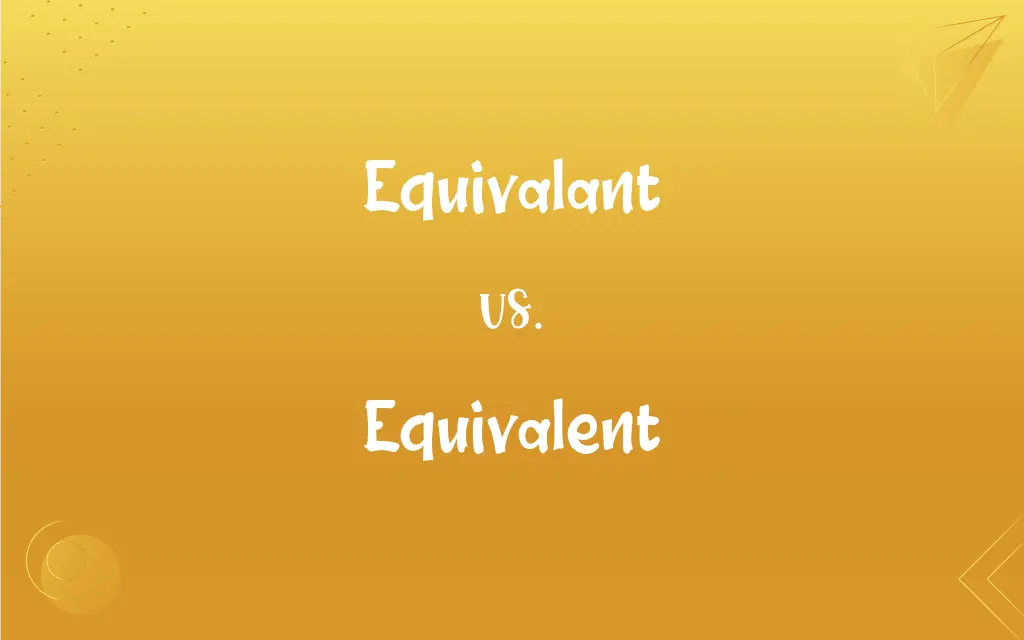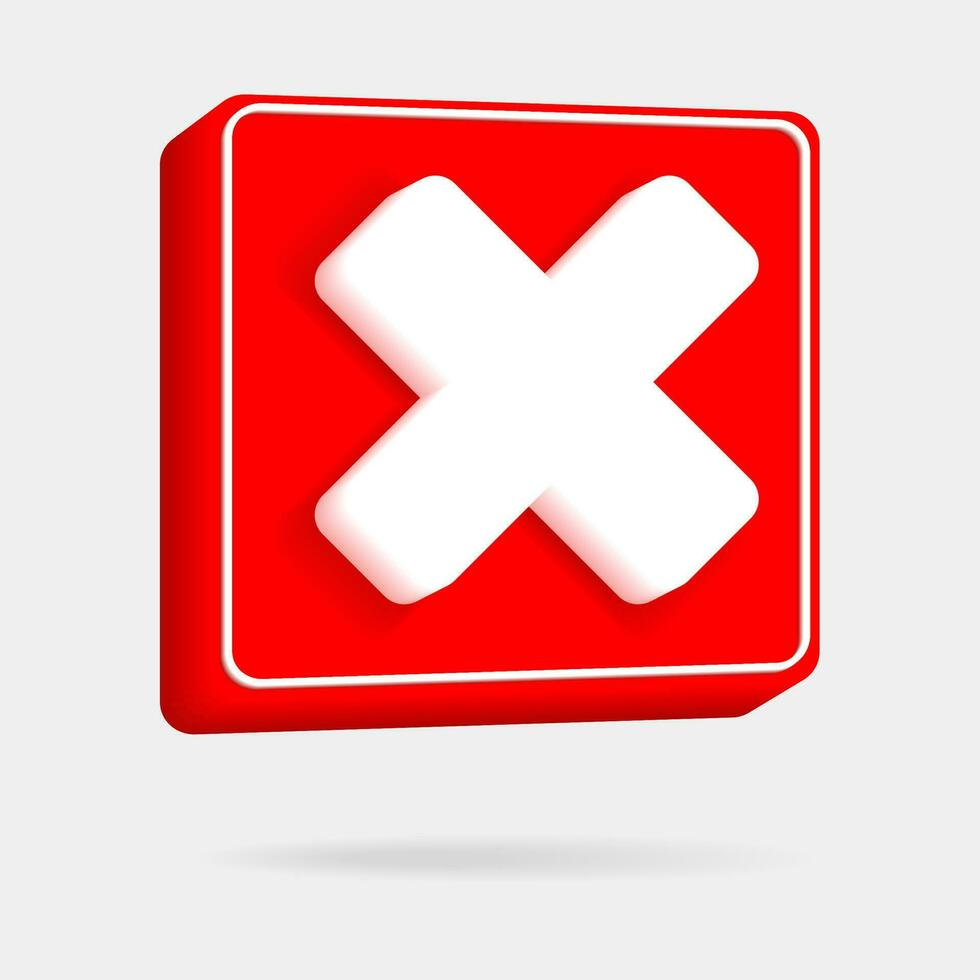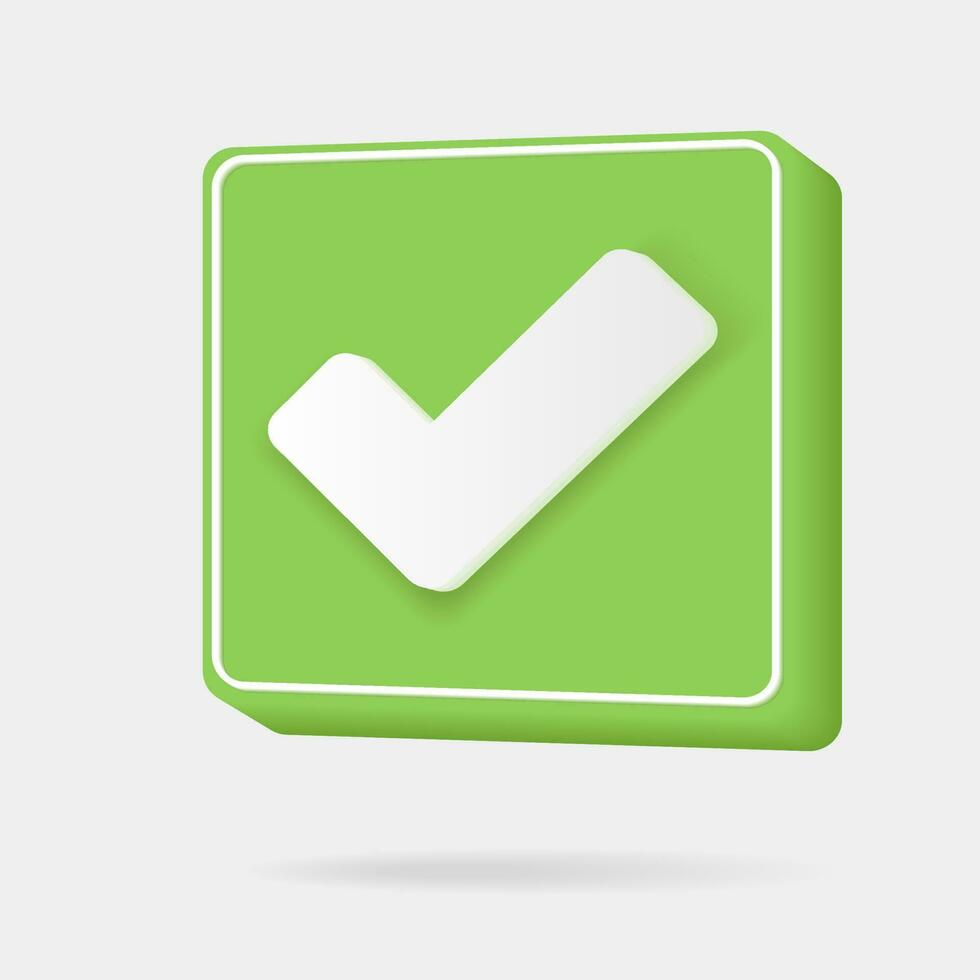# Equivalant vs. Equivalent: What's the Difference?Edited by Huma Saeed || By Sawaira Riaz || Updated on October 2, 2023
Equivalant is an incorrect spelling, while Equivalent is correct. Equivalent means having the same value, amount, function, or meaning.## Which is correct: Equivalant or Equivalent

How to spell Equivalent?### Equivalant is Incorrect## Key Differences

Focus on the "ent" ending in "Equivalent" similar to "different" and "consequent."
Remember the word "equity" (meaning fairness or value) as a reminder for the "equi-" prefix in "Equivalent."
Think of "Equivalent" as equal + ant, where "ant" is consistent in spelling.
Associate "Equivalent" with mathematics, as it's commonly used in equations.
Visualize the phrase "equal value" when writing "Equivalent" to reinforce its meaning and spelling.

## Equivalant and Equivalent Definitions

#### Equivalant

Equivalant is an incorrect spelling of Equivalent.

#### Equivalent

In chemistry, Equivalent describes substances having the same ability to combine.
Different acids have specific equivalent weights.

#### Equivalent

Equivalent refers to items or ideas of equal value or significance.
The word couch is the American equivalent of the British sofa.

#### Equivalent

Equivalent can refer to items having a similar function or role.
An online e-book is an equivalent to a traditional paper book in content.

#### Equivalent

It can also mean something interchangeable in some context.
A generic drug is considered equivalent to a brand-name drug.

#### Equivalent

Equal, as in value, force, or meaning.

#### Equivalent

Having similar or identical effects.

#### Equivalent

Being essentially equal, all things considered
A wish that was equivalent to a command.

#### Equivalent

Capable of being put into a one-to-one relationship. Used of two sets.

#### Equivalent

Having virtually identical or corresponding parts.

#### Equivalent

Of or relating to corresponding elements under an equivalence relation.

#### Equivalent

(Chemistry) Having the same ability to combine.

#### Equivalent

(Logic) Having equivalence
Equivalent propositions.

#### Equivalent

Something that is essentially equal to another
"The hand is not the biological equivalent of a hammer or a screwdriver.
The hand is a multipurpose tool like a Swiss Army knife" (Jonathan Gottschall).

#### Equivalent

(Chemistry) Equivalent weight.

#### Equivalent

Similar or identical in value, meaning or effect; virtually equal.
To burn calories, a thirty-minute jog is equivalent to a couple of hamburgers.

#### Equivalent

(mathematics) Of two sets, having a one-to-one correspondence.

#### Equivalent

(mathematics) Relating to the corresponding elements of an equivalence relation.

#### Equivalent

(chemistry) Having the equal ability to combine.

#### Equivalent

(cartography) Of a map, equal-area.

#### Equivalent

(geometry) Equal in measure but not admitting of superposition; applied to magnitudes.
A square may be equivalent to a triangle.

#### Equivalent

Anything that is virtually equal to something else, or has the same value, force, etc.

#### Equivalent

(chemistry) An equivalent weight.

#### Equivalent

(transitive) To make equivalent to; to equal.

#### Equivalent

Equal in worth or value, force, power, effect, import, and the like; alike in significance and value; of the same import or meaning.
For now to serve and to minister, servile and ministerial, are terms equivalent.

#### Equivalent

Equal in measure but not admitting of superposition; - applied to magnitudes; as, a square may be equivalent to a triangle.

#### Equivalent

Contemporaneous in origin; as, the equivalent strata of different countries.

#### Equivalent

Something equivalent; that which is equal in value, worth, weight, or force; as, to offer an equivalent for damage done.
He owned that, if the Test Act were repealed, the Protestants were entitled to some equivalent. . . . During some weeks the word equivalent, then lately imported from France, was in the mouths of all the coffeehouse orators.

#### Equivalent

That comparative quantity by weight of an element which possesses the same chemical value as other elements, as determined by actual experiment and reference to the same standard. Specifically: (a) The comparative proportions by which one element replaces another in any particular compound; thus, as zinc replaces hydrogen in hydrochloric acid, their equivalents are 32.5 and 1. (b) The combining proportion by weight of a substance, or the number expressing this proportion, in any particular compound; as, the equivalents of hydrogen and oxygen in water are respectively 1 and 8, and in hydric dioxide 1 and 16.

#### Equivalent

A combining unit, whether an atom, a radical, or a molecule; as, in acid salt two or more equivalents of acid unite with one or more equivalents of base.

#### Equivalent

To make the equivalent to; to equal; equivalence.

#### Equivalent

A person or thing equal to another in value or measure or force or effect or significance etc;
Send two dollars or the equivalent in stamps

#### Equivalent

The atomic weight of an element that has the same combining capacity as a given weight of another element; the standard is 8 for oxygen

#### Equivalent

Equal in amount or value;
Like amounts
Equivalent amounts
The same amount
Gave one six blows and the other a like number
An equal number
The same number

#### Equivalent

Being essentially equal to something;
It was as good as gold
A wish that was equivalent to a command
His statement was tantamount to an admission of guilt

#### Equivalent

In mathematics, Equivalent means having the same worth or result.
Two fractions that simplify to the same number are equivalent.

## FAQs

#### Which vowel is used before Equivalent?

"An" is used, as in "an equivalent."

#### What is the pronunciation of Equivalent?

It's pronounced as /ɪˈkwɪv.əl.ənt/.

#### Why is it called Equivalent?

It derives from the Latin word "aequivalentem," meaning "of equal value or power."

#### What is the root word of Equivalent?

The root is "equi-" meaning equal, and "-valent" meaning worth or value.

#### Which preposition is used with Equivalent?

"To" is common, as in "equivalent to."

#### Which article is used with Equivalent?

"An" or "the", as in "an equivalent" or "the equivalent."

No, "Equivalent" is not an adverb.

"Equivalents."

Neutral.

#### Is Equivalent a noun or adjective?

"Equivalent" can be both a noun and an adjective.

#### What is the verb form of Equivalent?

There isn't a direct verb form for "Equivalent." However, "equate" is related.

#### Is Equivalent a vowel or consonant?

"Equivalent" is a word and contains both vowels and consonants.

#### What is a stressed syllable in Equivalent?

The second syllable "quiv."

#### What is the opposite of Equivalent?

Unequal or non-equivalent.

"Equivalent."

#### Which conjunction is used with Equivalent?

Any conjunction can be used depending on the context.

#### What is the second form of Equivalent?

The word "equivalent" does not have verb forms.

#### Which determiner is used with Equivalent?

Common determiners include "this", "that", "my", "the", and "an."

#### How is Equivalent used in a sentence?

"The foreign currency is equivalent to fifty U.S. dollars."

#### Is Equivalent an abstract noun?

Yes, when referring to a concept or idea.

#### Is the word Equivalent imperative?

No, it's not imperative.

E-quiv-a-lent.

#### What is another term for Equivalent?

Synonym, counterpart, or equal.

#### Is Equivalent a countable noun?

Yes, you can have one equivalent, two equivalents, etc.

No, it isn't.

#### Is the Equivalent term a metaphor?

Not inherently, but can be used metaphorically in certain contexts.

#### How many syllables are in Equivalent?

There are four syllables.

#### What is the first form of Equivalent?

The word "equivalent" does not have verb forms.

#### What is the third form of Equivalent?

The word "equivalent" does not have verb forms.